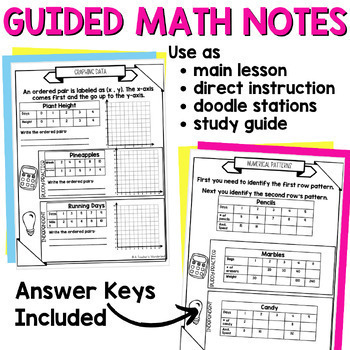# 5th Grade- Guided Math Notes Bundle - Test Prep - Printables - Math Practice

Rated 4.83 out of 5, based on 148 reviews
148 Ratings;
5th
Subjects
Resource Type
Formats Included
• Zip
Pages
100+
\$35.00
List Price:
\$44.00
You Save:
\$9.00
Bundle
\$35.00
List Price:
\$44.00
You Save:
\$9.00
Bundle
Report this resource to TPT

#### What educators are saying

These Math Notes are absolutely amazing! I tell everyone about them! My students love them and use them to look back on for review. This is absolutely fabulous.
Great math resource. love that this is a resource I can use for distance learning and for in-person learning. Used for spiral review but also great for dail notes in their IAN’s

#### Products in this Bundle (12)

showing 1-5 of 12 products

### Description

Do you ever teach a lesson and think it's amazing and then you get dozens of emails that the students didn't understand the lesson? Eliminate this problem using math notes. These math notes are great to use in your math class because they students will have a place to review the lesson.

How can you use this in your classroom?

•Direct Instruction

•Doodle Stations (complete part of the Math Notes during a specific rotation)

•Use as a study guide and for assessments

•Assign for homework

•Independent Time or after the lesson

Place Value & Multiplication

- Place Value

- Powers of ten & exponents

- Multiplication & Exponents

- Multiply 3 digits and 1 digit

-Multiplication strategies (3x2)

-Multiplication Strategies (3x2)

-Basic Multiplication strategies (3x2)

-PEMDAS

Division

-Place Value Patterns

- Relating Multiplication and Division

- Division with 1 digit divisor

- Divide by 2 digit divisors

- Estimate, divide by 2 digit divisors

- Partial Quotients

Division Models

- Remainders

- Place Value of Decimals

- Decimals to Thousandths

- Compare Decimals

- Rounding Decimals

-Decimal Subtraction using models

-Estimate Decimal Sums & Differences

Decimal Subtraction

Multiplying Decimals

- Multiplication Patterns with Decimals

- Multiply Decimals and Whole Numbers

- Multiplication Decimals and Whole Numbers

- Multiply using Expanded Form

-Multiplying Money Word Problems

-Decimal Multiplication

-Multiply Decimals: Place the Decimal Point

-Zeros in the product

Dividing Decimals

- Division Patterns with Decimals

- Divide Decimals by Whole Numbers

- Estimate Quotients

- Division of Decimals by Whole Numbers

- Decimal Division

-Multistep Decimal Problems

- Least Common Multiple

- Subtracting Unlike Denominators

-Subtracting Mixed Numbers

Multiplying Fractions

- Multiply by Whole Number

- Multiply by Whole Number

- Multiply by Whole Number by using one denominator

- Fraction by Fraction

- Greater than, Less than, or Equal to

-Simplest Form

- Multiply Mixed Numbers

- Multiply Mixed Numbers with Area Models

- Greater than, Less than, or Equal to

Dividing Fractions

- Connect Fractions to Division

- Divide Fractions by Whole Numbers

- Divide Whole Numbers by Fractions

- Word Problems

- Line Plots

Graphing

- Ordered Pairs

- Graph Data

- Numerical Patterns

- Graph & Analyze Relationships

- Graph and Analyze 2-Step Patterns(Problem Solving)

Conversions

- Customary Length

- Customary Capacity

- Customary Weight

- Customary Time

- Metric Conversions

Geometry & Volume

- Polygons

- Triangles

- Pyramids vs. Prisms

- Unit Cubes

- Volume

- Composite Figures

What does this include?

• 3 Different Templates Provided for each lesson
• Digital Version using. Google Slides

_____________________________________

Teachers like you said:

⭐️⭐️⭐️⭐️⭐️ I LOVE this resource. I often have students coming in and being pulled out for resource class so they often miss notes in my class. This resource has really made it SOOO much easier for all of my students to equally get all the information regardless of whether they were present or not.

⭐️⭐️⭐️⭐️⭐️I love that this is a resource I can use for distance learning and for in-person learning. Thank you for a thorough resource! It has really covered every topic I need.

⭐️⭐️⭐️⭐️⭐️kid-tested, teacher approved

_____________________________________

You may also like...

5th Grade- Guided Math Notes Bundle [Paper & Digital]

_____________________________________

Permission to copy for single classroom use only.

Total Pages
100+